### Home > CALC > Chapter 3 > Lesson 3.2.1 > Problem3-44

3-44.
1. Given the function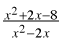, find the following limits without using your graphing calculator. Homework Help ✎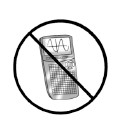1.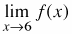2.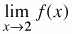3.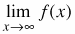4.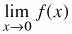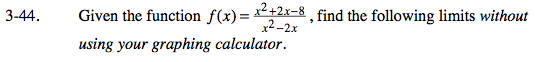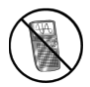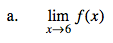Evaluate.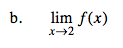Before finding the limit, factor the numerator and the denominator. If the '0' in the denominator cancels out, then the limit exists.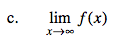For all limits as x →∞, you are looking for end behavior. For example, is there a horizontal asymptote? Or does the function approach +∞ or −∞ in the end? To answer this question, compare the highest power in the numerator with the highest power in the denominator.

Highest power in the numerator: x2

Highest power in the denominator: x2

$\lim_{x\rightarrow \infty }\frac{x^{2}}{x^{^{2}}}=\lim_{x\rightarrow \infty }1=1$

Note: this means that f(x) has a horizontal asymptote of y = 1.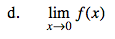First, factor the expression. Visualize the left-hand and right-hand limits of the simplified function. Do they match up?

You can prove this by showing that

$\lim_{x\rightarrow 0^{-1}}f(x)\neq \lim_{x\rightarrow 0^{+}}f(x).$

Limit does not exist.